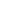# Free CBSE Class 9 Mathematics Unit 4-Geometry Triangles Worksheets

Download free printable Triangles Worksheets to practice. With thousands of questions available, you can generate as many Triangles Worksheets as you want.

## Sample CBSE Class 9 Mathematics Unit 4-Geometry Triangles Worksheet Questions

1.

State True/False: In triangles ABC and DEF, and AB = EF. The two triangles are congruent.

1. TRUE 2. FALSE
2.

State True/False: Angles opposite to equal sides of an isosceles triangle are equal.

1. TRUE 2. FALSE
3.

State True/False: Two triangles are congruent if two angles and the included side of one triangle are equal to two angles and the included side of other triangle.

1. TRUE 2. FALSE
4.

In  . Which side is hypotenuse?

1. AB 2. BC 3. AC 4. None of these
5.

Choose the correct option: In triangles ABC and DEF, AB = AC, and . The two triangles are ________.

1. isosceles and congruent 2. isosceles but not congruent 3. not isosceles but congruent 4. Neither isosceles nor congruent
6.

Choose the correct option: In triangles ABC and XYZ, BC = YZ and . The two triangles will be congruent by ASA axiom if _______.

1. Ã¢Â?Â A = Ã¢Â?Â X 2. Ã¢Â?Â B = Ã¢Â?Â X 3. Ã¢Â?Â C = Ã¢Â?Â Z 4. None of these
7.

Fill in the blanks: The value of each angle of an equilateral triangle is ________.

1. 30 Degrees 2. 45 Degrees 3. 90 Degree 4. 60 Degree
8.

Choose the correct option: If AB = QR, BC = PR and CA = PQ, then ________.

1. \Delta ABC \cong \Delta PQR 2. \Delta CBA \cong \Delta PRQ 3. \Delta BAC \cong \Delta RPQ 4. \Delta PQR \cong \Delta BCA
9.

Fill in the blanks: Hypotenuse of a right isosceles triangle is cm. The value of its side is ________.

1. 10 cm 2. 6 cm 3. 5 cm 4. 2.5 cm
10.

In  and . Which side is longest?

1. PR 2. PQ 3. QR 4. All are equal

Worksheets by UrbanPro

Our worksheets are designed to help students explore various topics, practice skills and enrich their subject knowledge, to improve their academic performance. Designed by Experts who have extensive experience and expertise in teaching a subject, these worksheets will improve your child's problem-solving skills and subject knowledge in a fun and interactive manner.
Check out our free customized worksheets across school boards, grades, subjects and levels of subject knowledge. You can download, print and share these worksheets with anyone, anywhere, anytime!

Get a custom worksheet to practice!

Select your topic & see the magic.

subjectSelect Chapter(s)

Chapters & Subtopics